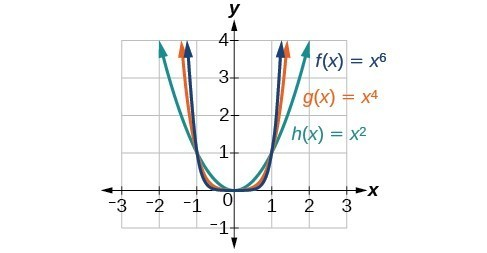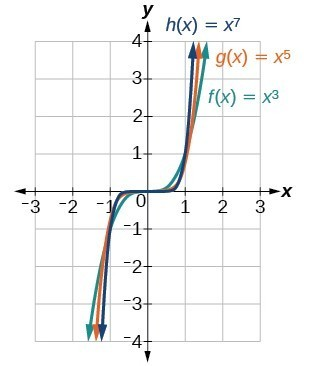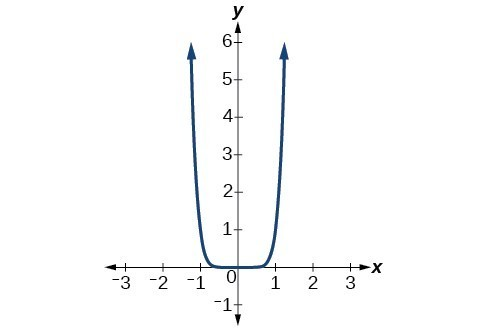## Describe the end behavior of power functions

### Identify power functions

In order to better understand the bird problem, we need to understand a specific type of function. A power function is a function with a single term that is the product of a real number, a coefficient, and a variable raised to a fixed real number. (A number that multiplies a variable raised to an exponent is known as a coefficient.)

As an example, consider functions for area or volume. The function for the area of a circle with radius is

$A\left(r\right)=\pi {r}^{2}$

and the function for the volume of a sphere with radius r is

$V\left(r\right)=\frac{4}{3}\pi {r}^{3}$

Both of these are examples of power functions because they consist of a coefficient, $\pi$ or $\frac{4}{3}\pi,$ multiplied by a variable r raised to a power.

### A General Note: Power Function

A power function is a function that can be represented in the form

$f\left(x\right)=k{x}^{p}$

where k and p are real numbers, and k is known as the coefficient.

### Q & A

Is $f\left(x\right)={2}^{x}$ a power function?

No. A power function contains a variable base raised to a fixed power. This function has a constant base raised to a variable power. This is called an exponential function, not a power function.

### Example 1: Identifying Power Functions

Which of the following functions are power functions?

$\begin{cases}f\left(x\right)=1\hfill & \text{Constant function}\hfill \\ f\left(x\right)=x\hfill & \text{Identify function}\hfill \\ f\left(x\right)={x}^{2}\hfill & \text{Quadratic}\text{ }\text{ function}\hfill \\ f\left(x\right)={x}^{3}\hfill & \text{Cubic function}\hfill \\ f\left(x\right)=\frac{1}{x} \hfill & \text{Reciprocal function}\hfill \\ f\left(x\right)=\frac{1}{{x}^{2}}\hfill & \text{Reciprocal squared function}\hfill \\ f\left(x\right)=\sqrt{x}\hfill & \text{Square root function}\hfill \\ f\left(x\right)=\sqrt{x}\hfill & \text{Cube root function}\hfill \end{cases}$

### Solution

All of the listed functions are power functions.

The constant and identity functions are power functions because they can be written as $f\left(x\right)={x}^{0}$ and $f\left(x\right)={x}^{1}$ respectively.

The quadratic and cubic functions are power functions with whole number powers $f\left(x\right)={x}^{2}$ and $f\left(x\right)={x}^{3}.$

The reciprocal and reciprocal squared functions are power functions with negative whole number powers because they can be written as $f\left(x\right)={x}^{-1}$ and $f\left(x\right)={x}^{-2}.$

The square and cube root functions are power functions with fractional powers because they can be written as $f\left(x\right)={x}^{1/2}$ or $f\left(x\right)={x}^{1/3}.$

### Try It 1

Which functions are power functions?

$\begin{cases}f\left(x\right)=2{x}^{2}\cdot 4{x}^{3}\hfill \\ g\left(x\right)=-{x}^{5}+5{x}^{3}-4x\hfill \\ h\left(x\right)=\frac{2{x}^{5}-1}{3{x}^{2}+4}\hfill \end{cases}$

Solution

### Identify end behavior of power functions

Figure 2 shows the graphs of $f\left(x\right)={x}^{2},g\left(x\right)={x}^{4}$ and $h\left(x\right)={x}^{6},$ which are all power functions with even, whole-number powers. Notice that these graphs have similar shapes, very much like that of the quadratic function in the toolkit. However, as the power increases, the graphs flatten somewhat near the origin and become steeper away from the origin.Figure 2. Even-power functions

To describe the behavior as numbers become larger and larger, we use the idea of infinity. We use the symbol $\infty$ for positive infinity and $-\infty$ for negative infinity. When we say that “x approaches infinity,” which can be symbolically written as $x\to \infty,\\$ we are describing a behavior; we are saying that x is increasing without bound.

With the even-power function, as the input increases or decreases without bound, the output values become very large, positive numbers. Equivalently, we could describe this behavior by saying that as $x$ approaches positive or negative infinity, the $f\left(x\right)$ values increase without bound. In symbolic form, we could write

$\text{as }x\to \pm \infty , f\left(x\right)\to \infty\\$

Figure 3 shows the graphs of $f\left(x\right)={x}^{3},g\left(x\right)={x}^{5},\text{and}h\left(x\right)={x}^{7},$ which are all power functions with odd, whole-number powers. Notice that these graphs look similar to the cubic function in the toolkit. Again, as the power increases, the graphs flatten near the origin and become steeper away from the origin.Figure 3. Odd-power function

These examples illustrate that functions of the form $f\left(x\right)={x}^{n}$ reveal symmetry of one kind or another. First, in Figure 2 we see that even functions of the form $f\left(x\right)={x}^{n}\text{, }n\text{ even,}$ are symmetric about the y-axis. In Figure 3 we see that odd functions of the form $f\left(x\right)={x}^{n}\text{, }n\text{ odd,}$ are symmetric about the origin.

For these odd power functions, as x approaches negative infinity, $f\left(x\right)$ decreases without bound. As x approaches positive infinity, $f\left(x\right)$ increases without bound. In symbolic form we write

$\begin{cases}\text{as } x\to -\infty , f\left(x\right)\to -\infty \\ \text{as } x\to \infty , f\left(x\right)\to \infty \end{cases}$

The behavior of the graph of a function as the input values get very small $(x\to -\infty)$ and get very large $(x\to \infty)$ is referred to as the end behavior of the function. We can use words or symbols to describe end behavior.

The table below shows the end behavior of power functions in the form $f\left(x\right)=k{x}^{n}$ where $n$ is a non-negative integer depending on the power and the constant.

### How To: Given a power function $f\left(x\right)=k{x}^{n}$ where n is a non-negative integer, identify the end behavior.

1. Determine whether the power is even or odd.
2. Determine whether the constant is positive or negative.
3. Use Figure 4 to identify the end behavior.

### Example 2: Identifying the End Behavior of a Power Function

Describe the end behavior of the graph of $f\left(x\right)={x}^{8}.$

### Solution

The coefficient is 1 (positive) and the exponent of the power function is 8 (an even number). As x approaches infinity, the output (value of $f\left(x\right)$ ) increases without bound. We write as $x\to \infty , f\left(x\right)\to \infty.$ As x approaches negative infinity, the output increases without bound. In symbolic form, as $x\to -\infty , f\left(x\right)\to \infty.\\$ We can graphically represent the function as shown in Figure 5.Figure 4

### Example 3: Identifying the End Behavior of a Power Function.

Describe the end behavior of the graph of $f\left(x\right)=-{x}^{9}.$

### Solution

The exponent of the power function is 9 (an odd number). Because the coefficient is –1 (negative), the graph is the reflection about the x-axis of the graph of $f\left(x\right)={x}^{9}.$ The graph shows that as x approaches infinity, the output decreases without bound. As x approaches negative infinity, the output increases without bound. In symbolic form, we would writeFigure 5. $\begin{cases}\text{as } x\to -\infty , f\left(x\right)\to \infty \\ \text{as } x\to \infty , f\left(x\right)\to -\infty \end{cases}$

### Analysis of the Solution

We can check our work by using the table feature on a graphing utility.

x f(x)
–10 1,000,000,000
–5 1,953,125
0 0
5 –1,953,125
10 –1,000,000,000

We can see from the table above that, when we substitute very small values for x, the output is very large, and when we substitute very large values for x, the output is very small (meaning that it is a very large negative value).

### Try It 2

Describe in words and symbols the end behavior of $f\left(x\right)=-5{x}^{4}.$

Solution